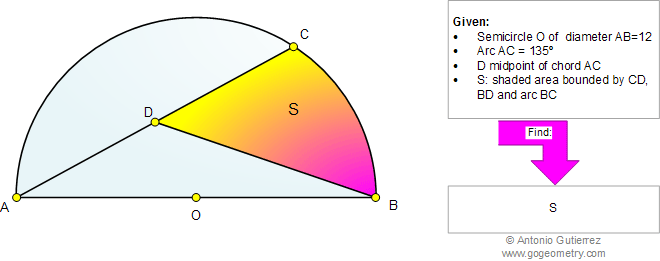# Online Geometry Problem 822: Circle, Semicircle, Arc, Chord, Midpoint, Sector, Triangle, Area. Level: High School, SAT Prep, College, Mathematics Education

 The figure below shows a semicircle O with diameter AB = 12. Arc AC = 135 degrees and D is the midpoint of chord AC. Find the shaded area bounded by CD, BD and arc BC.Geometry problem solving is one of the most challenging skills for students to learn. When a problem requires auxiliary construction, the difficulty of the problem increases drastically, perhaps because deciding which construction to make is an ill-structured problem. By “construction,” we mean adding geometric figures (points, lines, planes) to a problem figure that wasn’t mentioned as "given.

Home | SearchGeometry | Problems | All Problems | Open Problems | Visual Index | 10 Problems | 821-830 | Triangles | Circles | SemicircleAreas | Circular Sector | Midpoint | Email | Solution / comment | By Antonio Gutierrez